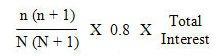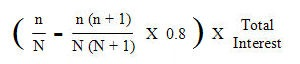# Car Loan Redemption Penalty

When signing up a car loan, typically you are subjected to a redemption penalty if you choose to redeem the loan before maturity. The redemption penalty is calculated based on the method of Rule of 78.

If you are interested in just getting a quick feel of how much redemption penalty you will have to pay on your car loan, skip to paragraph 5. Read on if you are interested in more background information.

According to the wikipedia entry on Rule of 78, apparently the number 78 might have come from the sum of numbers 1 to 12 (i.e. 1 + 2 + “¦ + 11 + 12 = 78). This method of calculating interest came about to simplify the calculation of the unearned portion of a loan’s interest in the pre-computer era, and continues to be used today for precomputed loans whereby the total interest chargeable on a loan is calculated and fixed before the loan is made, which is how car loans are made.

Here is how the rule of 78 works in a car loan. The rebate on unearned interest is calculated as:where n is the remaining number of months for the loan and N is the total number of months the loan period spans. The total interest is the interest rate multiplied by number of years of loan multiplied by loan amount.

This means that the loan redemption penalty is calculated as the remaining interest to be paid minus the rebate on unearned interest, which is as follows:Applying this formula, the following tables are derived showing the percentage of the total interest that will be charged as redemption penalty at the end of each year for 10, 7 and 5 years loan respectively.

 Yr 1 25.1% Yr 2 28.7% Yr 3 30.6% Yr 4 31.0% Yr 5 29.8% Yr 6 27.0% Yr 7 22.6% Yr 8 16.7% Yr 9 9.1% Yr 10 0%

 Yr 1 26.8% Yr 2 30.4% Yr 3 30.8% Yr 4 27.9% Yr 5 21.8% Yr 6 12.5% Yr 7 0%

 Yr 1 28.6% Yr 2 30.9% Yr 3 26.9% Yr 4 16.6% Yr 5 0%

As you can see, the redemption penalty is steep. Worse still, it doesn’t reward you for staying on longer with the loan. The bank and car sales agent is guaranteed to earn a commission of close to 30% of the total loan interest the moment you sign on the dotted line. Go figure.

(Visited 183 times, 1 visits today)
Posted inUncategorizedTags: